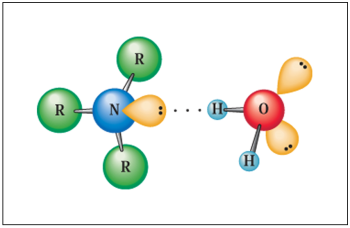Search:

# The physical properties of amines

- Images are due to Georgia Perimeter College -

## The $N-H$ bond

The electronegativity difference between $N$ and $H$ is low ($\Delta (E_n)$ $=$ $0,9$) compared for example to that between $O$ and $H$ ($\Delta (E_n)$ $=$ $1,4$).

## VolatilityThe weak hydrogen bonds between primary and secondary amine molecules are such that their volatility is less than that of alkanes, but greater than that of the corresponding alcohols:Thus, methylamine and ethylamine are gases at normal temperature, the methanol and ethanol not.

## SolubilityPrimary and secondary amines can establish hydrogen bonds with water molecules, hence their solubility in water up to $C_5$ terms.